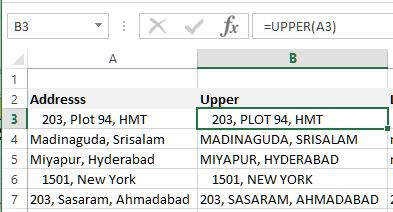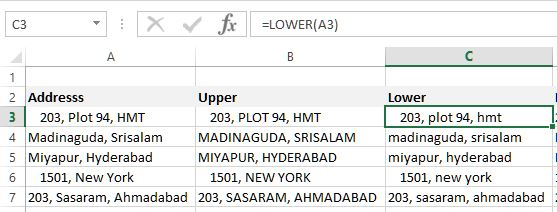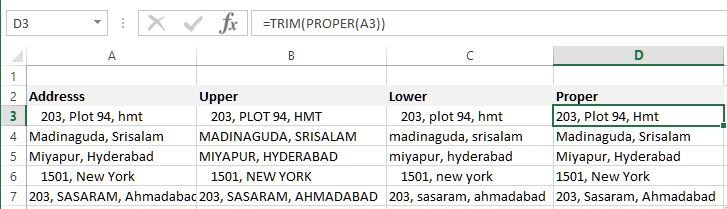# Upper/Lower/Proper case in Excel

##### How to change string to upper/lower/proper case in Excel?

In the previous post we learnt about how to count number of words in Excel. In this post, we shall learn how to change the case of a string (upper, lower or proper case) in Excel.

### UPPER function

To change string to upper case, we can use UPPER function. In B3 cell, we have '=UPPER(A3)' formula that changes the A3 cell string to upper case.

Here is the result.### LOWER function

To change string to lower case, we can use LOWER function. In C3 cell, we have '=LOWER(A3)' formula that changes the A3 cell string to lower case.

Here is the result.### PROPER function

To change string Proper case (1st character of each word will be in capital letter), we can use PROPER function. In D3 cell, we have written '=TRIM(PROPER(A3))' that first removes empty space from start and end of the string and then changes the string into Proper Case.

Here is the result.Views: 3178 | Post Order: 25